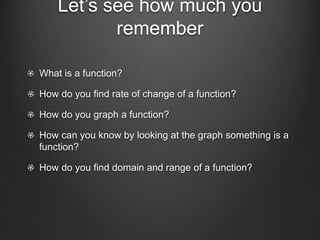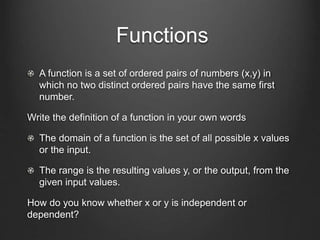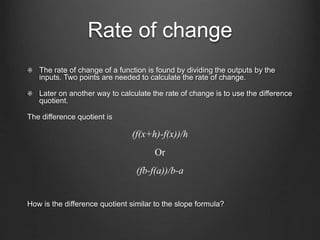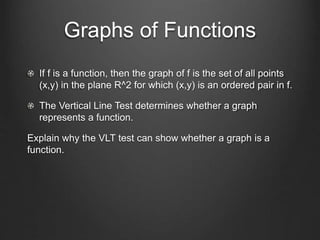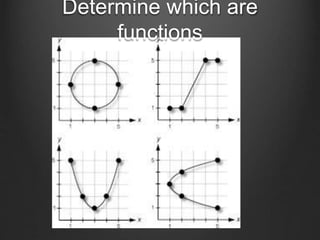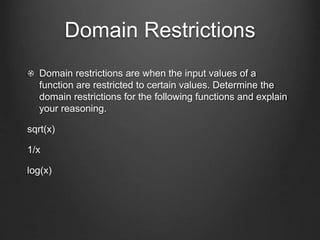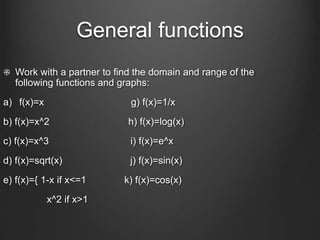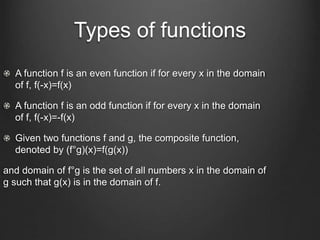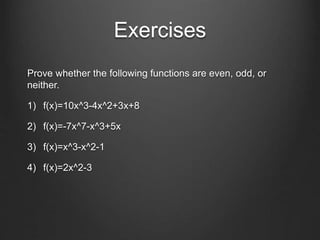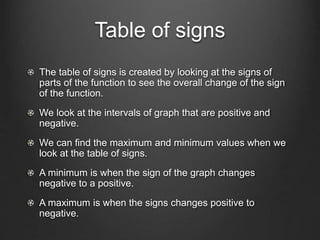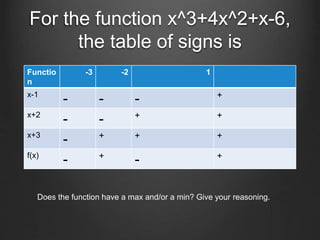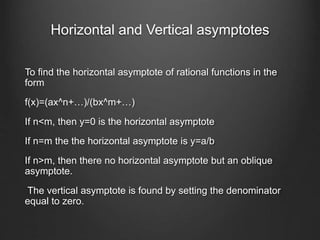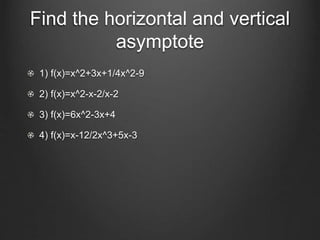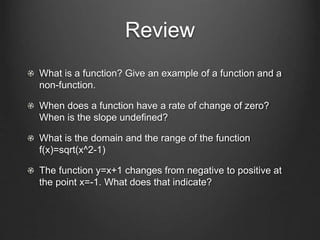1 de 15

### Edsc 304 lesson 1

1. Functions Review
2. Let’s see how much you remember What is a function? How do you find rate of change of a function? How do you graph a function? How can you know by looking at the graph something is a function? How do you find domain and range of a function?
3. Functions A function is a set of ordered pairs of numbers (x,y) in which no two distinct ordered pairs have the same first number. Write the definition of a function in your own words The domain of a function is the set of all possible x values or the input. The range is the resulting values y, or the output, from the given input values. How do you know whether x or y is independent or dependent?
4. Rate of change The rate of change of a function is found by dividing the outputs by the inputs. Two points are needed to calculate the rate of change. Later on another way to calculate the rate of change is to use the difference quotient. The difference quotient is (f(x+h)-f(x))/h Or (fb-f(a))/b-a How is the difference quotient similar to the slope formula?
5. Graphs of Functions If f is a function, then the graph of f is the set of all points (x,y) in the plane R^2 for which (x,y) is an ordered pair in f. The Vertical Line Test determines whether a graph represents a function. Explain why the VLT test can show whether a graph is a function.
6. Determine which are functions
7. Domain Restrictions Domain restrictions are when the input values of a function are restricted to certain values. Determine the domain restrictions for the following functions and explain your reasoning. sqrt(x) 1/x log(x)
8. General functions Work with a partner to find the domain and range of the following functions and graphs: a) f(x)=x g) f(x)=1/x b) f(x)=x^2 h) f(x)=log(x) c) f(x)=x^3 i) f(x)=e^x d) f(x)=sqrt(x) j) f(x)=sin(x) e) f(x)={ 1-x if x<=1 k) f(x)=cos(x) x^2 if x>1
9. Types of functions A function f is an even function if for every x in the domain of f, f(-x)=f(x) A function f is an odd function if for every x in the domain of f, f(-x)=-f(x) Given two functions f and g, the composite function, denoted by (f°g)(x)=f(g(x)) and domain of f°g is the set of all numbers x in the domain of g such that g(x) is in the domain of f.
10. Exercises Prove whether the following functions are even, odd, or neither. 1) f(x)=10x^3-4x^2+3x+8 2) f(x)=-7x^7-x^3+5x 3) f(x)=x^3-x^2-1 4) f(x)=2x^2-3
11. Table of signs The table of signs is created by looking at the signs of parts of the function to see the overall change of the sign of the function. We look at the intervals of graph that are positive and negative. We can find the maximum and minimum values when we look at the table of signs. A minimum is when the sign of the graph changes negative to a positive. A maximum is when the signs changes positive to negative.
12. For the function x^3+4x^2+x-6, the table of signs is Functio n -3 -2 1 x-1 - - - + x+2 - - + + x+3 - + + + f(x) - + - + Does the function have a max and/or a min? Give your reasoning.
13. Horizontal and Vertical asymptotes To find the horizontal asymptote of rational functions in the form f(x)=(ax^n+…)/(bx^m+…) If n<m, then y=0 is the horizontal asymptote If n=m the the horizontal asymptote is y=a/b If n>m, then there no horizontal asymptote but an oblique asymptote. The vertical asymptote is found by setting the denominator equal to zero.
14. Find the horizontal and vertical asymptote 1) f(x)=x^2+3x+1/4x^2-9 2) f(x)=x^2-x-2/x-2 3) f(x)=6x^2-3x+4 4) f(x)=x-12/2x^3+5x-3
15. Review What is a function? Give an example of a function and a non-function. When does a function have a rate of change of zero? When is the slope undefined? What is the domain and the range of the function f(x)=sqrt(x^2-1) The function y=x+1 changes from negative to positive at the point x=-1. What does that indicate?